A Survey of Gaussian Convolution Algorithms
Deriche Gaussian convolution

An accurate recursive filter approximation, computed out-of-place. More...

## Detailed Description

An accurate recursive filter approximation, computed out-of-place.

Deriche uses a sum of causal and an anticausal recursive filters to approximate the Gaussian. The causal filter has the form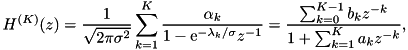where K is the filter order (2, 3, or 4). The anticausal form is the spatial reversal of the causal filter minus the sample at n = 0,The process to use these functions is the following:

1. deriche_precomp() to precompute coefficients for the convolution
2. deriche_gaussian_conv() or deriche_gaussian_conv_image() to perform the convolution itself (may be called multiple times if desired)
Example
buffer = (num *)malloc(sizeof(num) * 2 * N);
free(buffer);
Note
When the num typedef is set to single-precision arithmetic, deriche_gaussian_conv() may be inaccurate for large values of sigma.
Reference

## Data Structures

struct  deriche_coeffs
Coefficients for Deriche Gaussian approximation. More...

## Macros

#define DERICHE_MIN_K   2
Minimum Deriche filter order.

#define DERICHE_MAX_K   4
Maximum Deriche filter order.

#define DERICHE_VALID_K(K)   (DERICHE_MIN_K <= (K) && (K) <= DERICHE_MAX_K)
Test whether a given K value is a valid Deriche filter order.

## Typedefs

typedef struct deriche_coeffs deriche_coeffs
Coefficients for Deriche Gaussian approximation. More...

## Functions

static void make_filter (num *result_b, num *result_a, const complex *alpha, const complex *beta, int K, double sigma)
Make Deriche filter from alpha and beta coefficients. More...

void deriche_precomp (deriche_coeffs *c, double sigma, int K, num tol)
Precompute coefficients for Deriche's Gaussian approximation. More...

void deriche_gaussian_conv (deriche_coeffs c, num *dest, num *buffer, const num *src, long N, long stride)
Deriche Gaussian convolution. More...

void deriche_gaussian_conv_image (deriche_coeffs c, num *dest, num *buffer, const num *src, int width, int height, int num_channels)
Deriche Gaussian 2D convolution. More...

## Typedef Documentation

 typedef struct deriche_coeffs deriche_coeffs

Coefficients for Deriche Gaussian approximation.

The deriche_coeffs struct stores the filter coefficients for the causal and anticausal recursive filters of order K. This struct allows to precompute these filter coefficients separately from actually performing the filtering so that filtering may be performed multiple times using the same precomputed coefficients.

This coefficients struct is precomputed by deriche_precomp() and then used by deriche_gaussian_conv() or deriche_gaussian_conv_image().

## Function Documentation

 void deriche_gaussian_conv ( deriche_coeffs c, num * dest, num * buffer, const num * src, long N, long stride )

Deriche Gaussian convolution.

Parameters
 c coefficients precomputed by deriche_precomp() dest output convolved data buffer workspace array with space for at least 2 * N elements src input, overwritten if src = dest N number of samples stride stride between successive samples

This routine performs Deriche's recursive filtering approximation of Gaussian convolution. The Gaussian is approximated by summing the responses of a causal filter and an anticausal filter. The causal filter has the form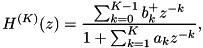where K is the filter order (2, 3, or 4). The anticausal form is the spatial reversal of the causal filter minus the sample at n = 0,The filter coefficients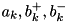correspond to the variables c.a, c.b_causal, and c.b_anticausal, which are precomputed by the routine deriche_precomp().

The convolution can be performed in-place by setting src = dest (the source array is overwritten with the result). However, the buffer array must be distinct from src.

Note
When the num typedef is set to single-precision arithmetic, results may be inaccurate for large values of sigma.

Definition at line 211 of file gaussian_conv_deriche.c.

 void deriche_gaussian_conv_image ( deriche_coeffs c, num * dest, num * buffer, const num * src, int width, int height, int num_channels )

Deriche Gaussian 2D convolution.

Parameters
 c coefficients precomputed by deriche_precomp() dest output convolved data buffer array with at least 2*max(width,height) elements src input image, overwritten if src = dest width image width height image height num_channels number of image channels

Similar to deriche_gaussian_conv(), this routine approximates 2D Gaussian convolution with Deriche recursive filtering.

The convolution can be performed in-place by setting src = dest (the source array is overwritten with the result). However, the buffer array must be distinct from src.

Note
When the num typedef is set to single-precision arithmetic, results may be inaccurate for large values of sigma.

Definition at line 347 of file gaussian_conv_deriche.c.

 void deriche_precomp ( deriche_coeffs * c, double sigma, int K, num tol )

Precompute coefficients for Deriche's Gaussian approximation.

Parameters
 c deriche_coeffs pointer to hold precomputed coefficients sigma Gaussian standard deviation K filter order = 2, 3, or 4 tol accuracy for filter initialization at the boundaries

This routine precomputes the recursive filter coefficients for Deriche's Gaussian convolution approximation. The recursive filters are created through the following steps:

1. First, the causal filter is represented in the form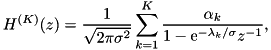where the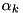and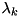are Deriche's optimized parameters and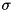is the specified sigma value.
2. Setting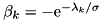, the filter is algebraically rearranged by make_filter() to the form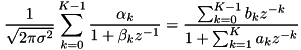to obtain the numerator and denominator coefficients for the causal filter.
3. The anticausal filter is determined according to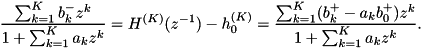Definition at line 62 of file gaussian_conv_deriche.c.

 static void make_filter ( num * result_b, num * result_a, const complex * alpha, const complex * beta, int K, double sigma )
static

Make Deriche filter from alpha and beta coefficients.

Parameters
 result_b resulting numerator filter coefficients result_a resulting denominator filter coefficients alpha,beta input coefficients K number of terms sigma Gaussian sigma parameter

This routine performs the algebraic rearrangementto obtain the numerator and denominator coefficients for the causal filter in Deriche's Gaussian approximation.

The routine initializes b/a as the 0th term,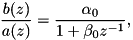then the kth term is added according to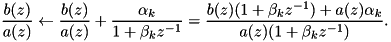Definition at line 145 of file gaussian_conv_deriche.c.